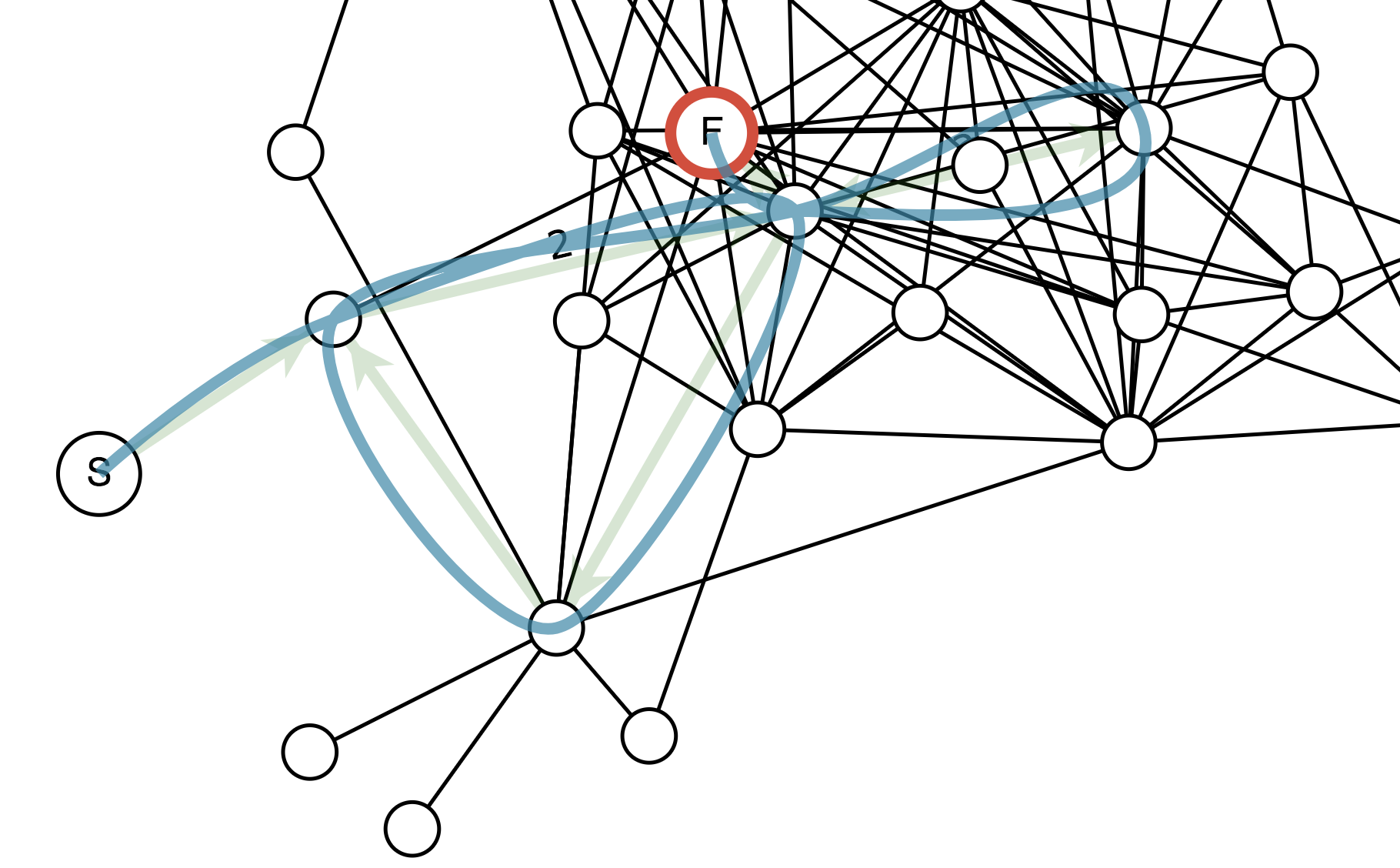Nobody Packages More

# npm

## d3-force-straighten-paths

1.0.2 • Public • Published

# d3-force-straighten-pathsGiven a set of paths as arrays of node ids or references, and the strength associated with each path, apply torsion spring forces to each node to attempt to straighten the paths.

This helps with drawing paths (chains of edges) as splines. The torsion forces are applied each time there is an angle between two consecutive edges in a path:In the diagram, arrows represent the forces on three consecutive nodes in a chain. Nodes A and C are pushed outward perpendicular to their respective edges to B. And B is brought between A and C with a force equal to the negative sum of the other two forces.

The force is proportional to the square of the difference between ∠B and π—this penalizes tight angles more than angles closer to straight.

## API Reference

# forceStraightenPaths([paths])

Creates a new force with the given array of paths. If paths is not specified, it defaults to the empty array.

# straighten.paths([paths])

If paths is specified, sets the force's paths to the specified array of objects. For each path object, straighten.pathNodes will be called to get the array of nodes, and straighten.pathStrength will be called to get the multiplier for the torsion strength of each of the internal nodes on the path.

The paths array is not modified by `forceStraightenPaths`. If the nodes arrays contain objects, they are assumed to be references to the nodes of the simulation. Otherwise straighten.id is used to build a map of the nodes, and the nodes are used as keys into that map to get node references.

# straighten.id([id])

The function to retrieve a key for each node used to identify that node. straighten.pathNodes should return an array of compatible keys. Defaults to fetching node.index.

# straighten.angleForce([angleForce])

The constant to be multiplied by the square of the difference of the angle from π to produce the force. Default: 0.1.

# straighten.pathNodes([pathNodes])

Given a path, fetches the array of nodes. Defaults to fetching path.nodes.

# straighten.pathStrength([pathStrength])

Given a path, determines the strength multiplier. Defaults to fetching path.strength, if it is defined, otherwise 1.

# straighten.debug([debug])

Debug mode. Prints output to the console for use with force-debugger.

## Keywords

### Install

`npm i d3-force-straighten-paths`

### Repository

github.com/att/d3-force-straighten-paths

### Homepage

github.com/att/d3-force-straighten-paths

327

1.0.2